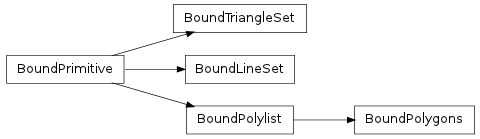#### Next topic

A triangle set bound to a transform matrix and materials mapping.__init__(ts, matrix, materialnodebysymbol)

Create a bound triangle set from a triangle set, transform and material mapping. This gets created when a triangle set is instantiated in a scene. Do not create this manually.

Methods

 __init__(ts, matrix, materialnodebysymbol) Create a bound triangle set from a triangle set, transform and material mapping. generateNormals() If normals is None or you wish for normals to be shapes() Iterate through all the triangles contained in the set. triangles() Iterate through all the triangles contained in the set.

Attributes

 normal Read-only numpy.array of size Nx3 where N is the number of normal values in the normal_index Read-only numpy.array of size Nx3 where N is the number of vertices in the primitive. texcoord_indexset Read-only tuple of texture coordinate index arrays. texcoordset Read-only tuple of texture coordinate arrays. vertex Read-only numpy.array of size Nx3 where N is the number of vertex points in the vertex_index Read-only numpy.array of size Nx3 where N is the number of vertices in the primitive.
normal

Read-only numpy.array of size Nx3 where N is the number of normal values in the primitive’s normal source array. The values will be transformed according to the bound transformation matrix.

normal_index

Read-only numpy.array of size Nx3 where N is the number of vertices in the primitive. To get the actual normal values, one can use this array to select into the normals array, e.g. normal[normal_index]. The values will be transformed according to the bound transformation matrix.

texcoord_indexset

Read-only tuple of texture coordinate index arrays. Each value is a numpy.array of size Nx2 where N is the number of vertices in the primitive. To get the actual texture coordinates, one can use the array to select into the texcoordset array, e.g. texcoordset[texcoord_indexset] would select the first set of texture coordinates. The values will be transformed according to the bound transformation matrix.

texcoordset

Read-only tuple of texture coordinate arrays. Each value is a numpy.array of size Nx2 where N is the number of texture coordinates in the primitive’s source array. The values will be transformed according to the bound transformation matrix.

vertex

Read-only numpy.array of size Nx3 where N is the number of vertex points in the primitive’s vertex source array. The values will be transformed according to the bound transformation matrix.

vertex_index

Read-only numpy.array of size Nx3 where N is the number of vertices in the primitive. To get the actual vertex points, one can use this array to select into the vertex array, e.g. vertex[vertex_index]. The values will be transformed according to the bound transformation matrix.

triangles()

Iterate through all the triangles contained in the set.